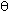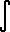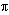Home    |    Teacher    |    Parents    |    Glossary    |    About UsEmail this page to a friendResources· Cool Tools · Formulas & Tables · References · Test Preparation · Study Tips · Wonders of MathSearchComplexity (Math | Miscellaneous | Complexity | ei theta)

### Justifications that ei= cos() + i sin()

ei x = COs( x ) + i sin( x )

### Justification #1: from the derivative

Consider the function on the right hand side (RHS)
f(x) = COs( x ) + i sin( x )

Differentiate this function
f ' (x) = -sin( x ) + i COs( x) = i f(x)

So, this function has the property that its derivative is i times the original function.
What other type of function has this property?

A function g(x) will have this property if
dg / dx = i g
This is a differential equation that can be solved with separation of variables
(1/g) dg = i dx(1/g) dg =i dx
ln| g | = i x + C
| g | = ei x + C = eC ei x
| g | = C2 ei x
g = C3 ei x

So we need to determine what value (if any) of the constant C3 makes g(x) = f(x).
If we set x=0 and evaluate f(x) and g(x), we get
f(x) = COs( 0 ) + i sin( 0 ) = 1
g(x) = C3 ei 0 = C3
These functions are equal when C3 = 1.

Therefore,
COs( x ) + i sin( x ) = ei x

### Justification #2: the series method

(This is the usual justification given in textbooks.)

By use of Taylor's Theorem, we can show the following to be true for all real numbers:
sin x = x - x3/3! + x5/5! - x7/7! + x9/9! - x11/11! + ...

COs x = 1 - x2/2! + x4/4! - x6/6! + x8/8! - x10/10! + ...

ex = 1 + x + x2/2! + x3/3! + x4/4! + x5/5! + x6/6! + x7/7! + x8/8! + x9/9! + x10/10! + x11/11! + ...
Knowing that, we have a mechanism to determine the value of ei, because we can express it in terms of the above series:
e^(i) = 1 + (i) + (i)2/2! + (i)3/3! + (i)4/4! + (i)5/5! + (i)6/6! + (i)7/7! + (i)8/8! + (i)9/9! + (i)10/10! + (i)11/11! + ...
We know how to evaluate an imaginary number raised to an integer power, which is done as such:
i1 = i
i2 = -1     terms repeat every four
i3 = -i
i4 = 1
i5 = i
i6 = -1
etc...
We can see that it repeats every four terms. Knowing this, we can simplify the above expansion:
e^(i) = 1 +i -2/2! - i3/3! +4/4! + i5/5! -6/6! - i7/7! +8/8! + i9/9! -10/10! - i11/11! + ...
It just so happens that this power series can be broken up into two very convenient series:

e^(i) =
[1 -2/2! +4/4! -6/6! +8/8! -10/10! + ...]
+
[i- i3/3! + i5/5! - i7/7! + i9/9! - i11/11! + ...]
Now, look at the series expansions for sine and cosine. The above above equation happens to include those two series. The above equation can therefore be simplified to
e^(i) = COs() + i sin()
An interesting case is when we set=, since the above equation becomes
e^(i) = -1 + 0i = -1.
which can be rewritten as
e^(i) + 1 = 0.     special case
which remarkably links five very fundamental constants of mathematics into one small equation.

Again, this is not necessarily a proof since we have not shown that the sin(x), COs(x), and ex series converge as indicated for imaginary numbers.